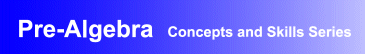Award-Winning

Pre-Algebra Interactive Learning Software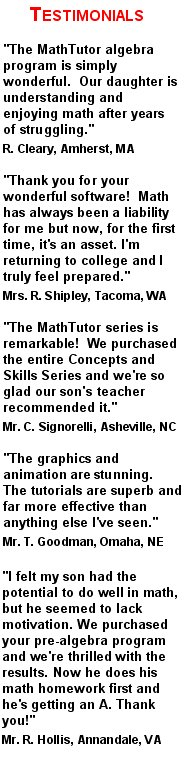DESCRIPTION
PRE-ALGEBRA is the ideal

software tutorial program for learning and mastering pre-algebra.

Students learn the concepts of pre-algebra, build problem-solving skills, and build a solid foundation for further math learning.

PRE-ALGEBRA is a dynamic interactive learning program designed for students at all levels of ability.

Students receive personalized on-demand coaching and hints as they learn.
Parents seeking homeschool pre-algebra tutorial and learning software will find the program to be perfectly suited to their needs.
Schools seeking

curriculum-based pre-algebra teaching and review software

will find the program to be ideal.
PRE-ALGEBRA is research-based and has been acclaimed for its educational excellence as well as its flexibility. It can be used for self-study and to supplement classroom instruction in high school and middle school math classes. The program also contains diagnostic and assessment tests that measure student skills and provide a study plan customized for each student.
Designed by math educators and in accordance with math curriculum standards, the program is also effective when used as software for remedial and developmental math teaching.
• Award-winning, curriculum-based pre-algebra courseware developed by math educators
• Provides dynamic, interactive pre-algebra lessons that are ideal for self-study, and as a supplement to classroom instruction
• Demonstrated success in boosting students' math skills and comprehension, and proficiency in problem solving
• Network versions contain student record-keeping, grading, and performance analysis features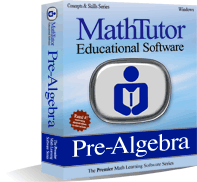TOPICS COVERED
• Place Value
• Ordering Numbers
• Rounding Numbers
• Subtracting Whole Numbers
• Word Problems
Multiplying and Dividing Whole Numbers
• Multiplying By 1-Digit Numbers
• Multiplying By Whole Numbers
• Dividing By 1 and 2 Digit Numbers
• Dividing By Whole Numbers
• Word Problems
Other Operations Using Whole Numbers
Adding and Subtracting Fractions and Mixed Numbers
• Fractions and Mixed Numbers
• Finding Equivalent Fractions
• Reducing Fractions to Lowest Terms
• Comparing and Ordering Fractions
• Adding Fractions and Mixed Nos.
• Subtracting Fractions
• Subtracting Fractions and Mixed Nos.
Multiplying and Dividing Fractions and Mixed Numbers
• Multiplying Fractions
• Multiplying Fractions and Mixed Nos.
• Dividing Fractions
• Dividing Fractions and Mixed Nos.
• Word Problems
Operations With Decimals
• Place Value and Decimal Numbers
• Comparing and Rounding Decimal Nos.
• Subtracting Decimal Numbers
• Multiplying Decimal Numbers
• Dividing Decimal Numbers
• Word Problems
Positive and Negative Numbers
• The Number Line
• Subtraction
• Multiplication
• Division
• Positive and Negative Exponents
• Scientific Notation
Expressions and Formulas
• Variables and Expressions
• Like Terms
• Simplifying Expressions
Equations
Percent
Ratio and Proportion
• Ratios and Rates
• Solving Proportions
• Measurement
• Word Problems
Inequalities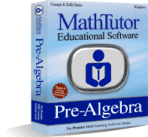Order Now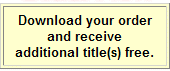TOPICS COVERED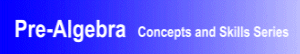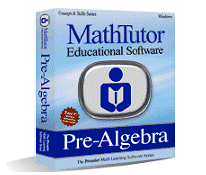TOPICS COVERED